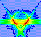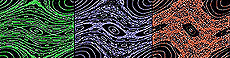Main Page | What is Chaos?The Standard Map: Quick Introduction The Standard Map is the name of a specific two-dimensional dynamical map that is useful for studying the basic features of chaotic motion. A "map" in this sense means that it is a pair of mathematical transformation equations that can be plotted on a two-dimensional graph. As in all dynamical maps that physicists study, the action of the map represents the evolution of a real physical system in time. In particular, the Standard Map is a two-dimensional coupled map in phase space (You can learn what this means from the tutorial). The physical system represented by the map is called the kicked rotor. A "rotor" in this sense can be imagined as a bead sliding in a frictionless manner around on a circular wire. The "kicked" part comes from the fact that the rotor is subjected to a "driving force" that comes at exactly even intervals in time. In physical terms, the kick might be delivered by an electromagnetic pulse, for example. To make things as simple as possible, the driving force (that is, the kick) always arrives from the same direction in space, and with exactly the same intensity. The driving force either speeds up or slows down the bead, depending on where the bead happens to be at the moment the kick arrives. In other words, even though the intensity of the kick is always the same, and it always comes from the same direction in space, the amount of the kick that is absorbed by the bead in the form of a change of speed depends on the position of the bead around the circle at that instant. The relationship is expressed mathematically through the equations of the map. On the plot of the Standard Map, the horizontal axis represents the position of the bead around the circle at the moment the kick arrives. The vertical axis represents the angular speed at the same moment. This type of position-speed plot is called phase space by physicists, and it is a very powerful tool for studying chaos. The most interesting aspect of the Standard Map is not simply that it shows chaos, but that it shows the most basic minimalist conditions under which chaos can occur. Chaotic motion in the map is shown by the "mixing up" of initial conditions. In the applet here, different initial conditions are shown in different colors. Chaotic motion is indicated by the mixing up of colors. As it happens, if the kick strength happens to be set to a low value, the system is not chaotic at all. At some intermediate values, the system is chaotic for some initial conditions, but not for others. If the driving strength increased, more and more initial conditons are chaotic. Yet even at high driving strength, there still remain initial conditons which are not chaotic. You can explore the effect of different driving strengths on the appearance of the map. It has been established that the onset of chaotic trajectories occur at a dimensionless driving strength of about 0.71. Below the critical value, no chaotic trajectories exist, and thus the initial conditions remain segregated from each other as the map as interated. Just above the critical value, the first chaotic trajectories appear, as can be seen by the mixing up of colors.